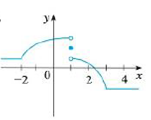Chapter 2.8, Problem 44E

Chapter
Section
Textbook Problem

The graph of f is given. State, with reasons, the numbers at which f is not differentiable.To determine

To state: The graph of the function f is not differentiable at which the numbers.

Explanation

Note:

The function f is not differentiable at the point a, then it must be satisfied any of the following conditions.

(i) The function f is discontinuous at the point a.

(ii) The function f has a corner point at the point a.

(iii) The function f has a vertical tangent at the point a.

From the graph of f, it is observed that f has a corner points at x=2 and x=3

Still sussing out bartleby?

Check out a sample textbook solution.

See a sample solution

The Solution to Your Study Problems

Bartleby provides explanations to thousands of textbook problems written by our experts, many with advanced degrees!

Get Started

Evaluate the integral. 1tan1+tand

Calculus (MindTap Course List)

In Exercises 7-28, perform the indicated operations and simplify each expression. 11. 583(3t+2)+13

Applied Calculus for the Managerial, Life, and Social Sciences: A Brief Approach

Evaluate the integral. 022x1dx

Calculus: Early Transcendentals

90kg=lb

Elementary Technical Mathematics

The graph at the right has equation:

Study Guide for Stewart's Multivariable Calculus, 8th

The average value of f(x) = 3x2 + 1 on the interval [2, 4] is: a) 29 b) 66 c) 58 d) 36

Study Guide for Stewart's Single Variable Calculus: Early Transcendentals, 8th